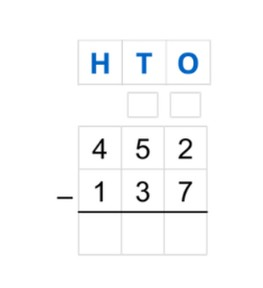Subtraction to 1,000 using standard algorithm with regrouping

# Subtraction to 1,000 using standard algorithm with regrouping

Students subtract numbers to 1,00 using standard algorithm with regrouping.8,000 schools use Gynzy92,000 teachers use Gynzy1,600,000 students use Gynzy

## General

Students learn to subtract numbers to 1,000 using the standard algorithm crossing tens and hundreds, using the terminology of "borrowing" from the hundreds or tens.

4.NBT.B.4

## Relevance

By learning how to subtract using the standard algorithm, students are able to more easily solve subtraction problems with larger numbers.

## Introduction

Ask the students to create the given numbers by dragging the MAB blocks. Have them name how many hundreds, tens, and ones are required to create the number.

## Development

Explain to students that when using the standard algorithm, we can more easily solve subtraction problems. Remind students that H stands for Hundreds, T stands for Tens, and O stands for Ones. Show students step by step how to solve using the standard algorithm. Ask students if they remember what the little boxes above the HTO stand for (they are used when regrouping). The problem 452 - 137 is written one below the other, in the place value chart. Solve from right to left. You first subtract the ones. The ones number on the bottom is greater than the ones number on the top. This means that you need to borrow ten from the tens column. You do so by crossing out the 5 and writing 4 in the little box. You then add the ten to your ones number, which makes it 12. You then subtract 12 - 7 for the ones column. Next you subtract the tens, and remember to subtract using the number in the little box, not the number you crossed out. Finally you subtract the hundreds and you have solved the problem using the standard algorithm. With the next problems, ask students to determine what the difference is for the yellow boxes. Next they practice a subtraction problem in which they must regroup the tens by borrowing from the hundreds number. Have students work through this problem with you on the board. Then give a few practice problems to solve individually or in pairs.

Check that students understand subtracting numbers to 1,000 using the standard algorithm with regrouping by asking the following questions:
- Why is it useful to be able to use the standard algorithm?
- What do the letters H, T, and O stand for?
- Where do you start subtracting when using the standard algorithm?
- Solve the following problems using the standard algorithm: 458 - 129 = ? and 763 - 382 = ?

## Guided practice

Students are given subtraction problems in which they must solve their problems in the place value chart. They are given problems in which they must borrow from the tens column as well as from the hundreds column. They must also write the subtraction problems in the place value chart and then solve for the difference.

## Closing

Ask students to come up with problems on their own by dragging numbers to the place value chart. Make sure that different kinds of problems are produced: borrowing from the tens, and borrowing from the hundreds. Discuss why it is useful to be able to use the standard algorithm and explain how this is done. Remind students that you always start on the right and borrow from the neighbor column when the bottom number is greater than the top number. You learn how to more easily subtract larger numbers.

## Teaching tips

To support students who have difficulty with the standard algorithm, start by working on their knowledge of the place value chart (HTO) and how to correctly write a number into the place value chart. You can choose to write the subtraction problems of the ones, tens, hundreds separately and then to write those differences together to make it extra clear which numbers are being subtracted. For students who have difficulty understanding the borrowing(or regrouping), they can be supported by making use of MAB blocks to physically move blocks from the tens column to the ones column.

## Instruction materials

Optional: MAB blocks.

### The online teaching platform for interactive whiteboards and displays in schools

• Save time building lessons

• Manage the classroom more efficiently

• Increase student engagement